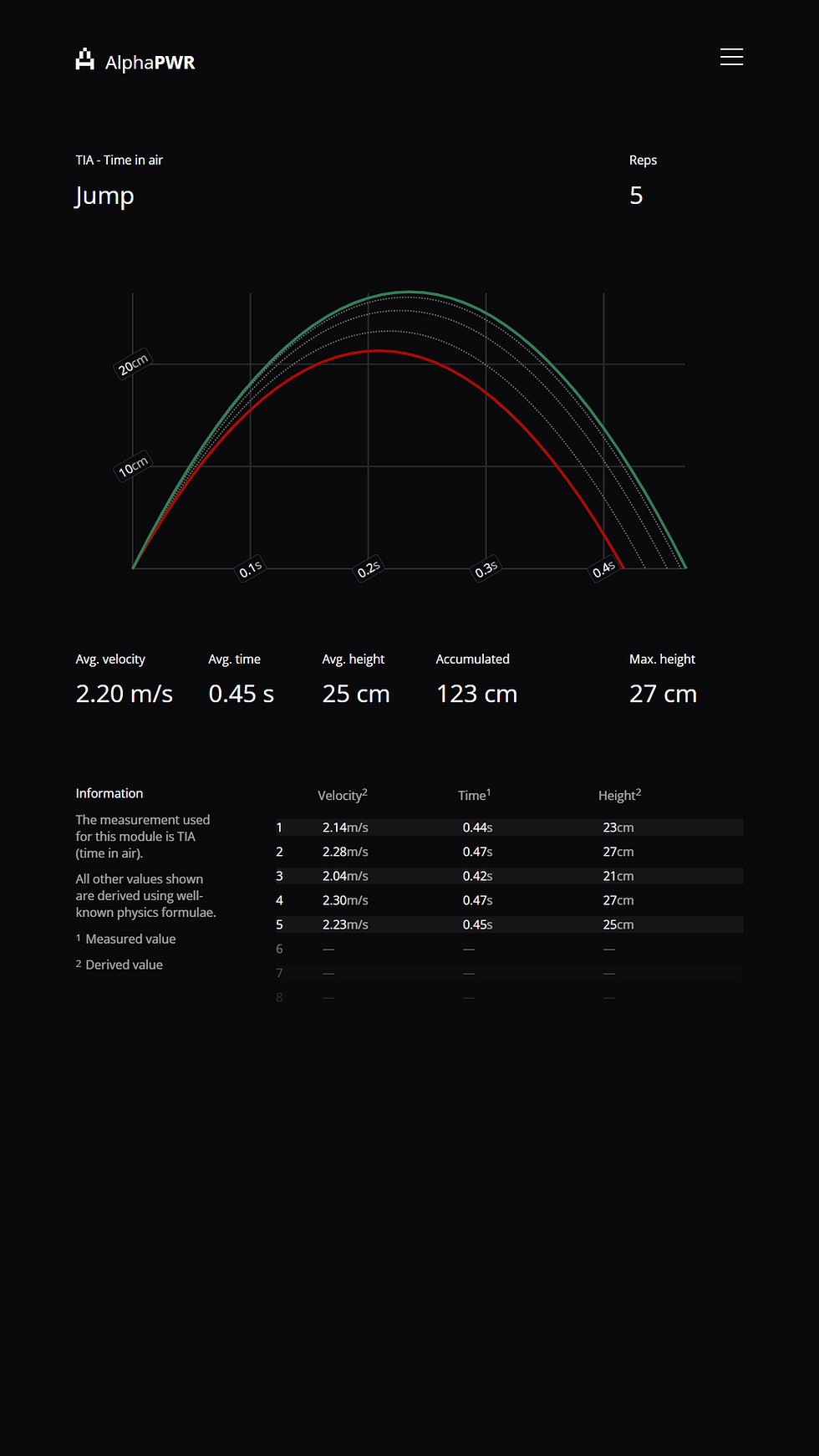top of page

# Jump Measurements

Oppdatert: 11. jul.

"all of the metrics (...) can be derived from only one (specifically any one) of them"

As you might have seen, selecting "Jump" in our remote control gives two choices for algorithms for measuring jumps. These are time in air (TIA) and take-off velocity (TOV). Before going into how these work, I'll try to explain the basics of jump metrics, as there are some points of potential confusion here.The most widely used metrics for describing a jump can be said to be take-off velocity, time in air, and height. Notice that both TOV and TIA are mentioned both as metrics and as algorithms, this duality should be understandable by the end of this article.

An important thing to understand is that all of the metrics mentioned above can be derived from only one (specifically any one) of them. For example, knowing the take-off velocity of a jump, we can trust our friend Newton, and use his laws of motion to calculate height and time in air. This is because as soon as your body leaves the forceplate, it can essentially be viewed as an object in free fall.

So, we now know that these three metrics can be derived from only one. Still, we need to measure one of them, which is where the algorithm/metric duality comes in. The TIA algorithm measures TIA first, and then derives TOV and height, while the TOV algorithm first measures TOV, then derives TIA and height.

#### TIA (time in air) algorithm

Time in air is very straight forward to measure with a force plate, as we can register the amount of time no force was registered on the plate, i.e. how long it took between take-off to landing. The simple and easy to understand nature of this algorithm has made it very popular, but it has a few drawbacks. If the user pulls up their legs during the jump and lands with bent knees, the measured time in air will be less indicative of the actual height and TOV than if they land with straight legs. In other words, to get reliable measurement using TIA, one has to also have reliable technique.

#### TOV (take-off velocity) algorithm

Measuring this metric is quite a bit more intricate than TIA, because a forceplate does not directly measure velocity. The algorithm will do its best to find the beginning of the users motion leading up to the jump, and then do numerical integration to get to velocity at the exact point of take-off. This eliminates the shortcomings of the TIA algorithm, but is prone to its own errors, mainly choosing a suboptimal point for starting the integration, and choosing the wrong point of take-off.